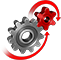# WolframSystemModeler

## Inverted Pendulum

Stabilize the unstable pendulum with a linear-quadratic regulator. This type of feedback regulator, which is often used to control robotics, is here used to keep an inverted pendulum upright.

To run this example, you'll need

### Inverted Pendulum Model

The inverted pendulum model consists of a pendulum and a cart, with the axis of rotation of the pendulum being located at the center of the cart. The pendulum is initialized with its center of mass above the rotation axis. When located directly above the cart, the pendulum will be in steady state and will stay there until disturbed.Animation of the inverted pendulum.
While a pendulum that hangs straight down will be in a stable position, an inverted pendulum is unstable. That means that any small disturbance will cause the pendulum to tip over and never return to its original position.

### Control System

A control system can be used to stabilize the unstable pendulum. Here, a linear-quadratic regulator is used. First, the regulator measures the states of the system, namely the cart position and velocity, the pendulum angle and the angular velocity. The regulator then calculates a force that should be applied on the cart in order for all the states to become zero. In other words, the regulator aims to have the pendulum pointing straight up (defined here as 0 degrees) and the cart return to the origin.

### External Connection

With the free ModelPlug library, an Arduino board can be connected to the model to allow setting the disturbance forces in real time by controlling the input to the two analog pins specified in the model. This can be done with thumbwheel potentiometers, for example.

## Wolfram System Modeler

Questions? Comments? Contact a Wolfram expert »